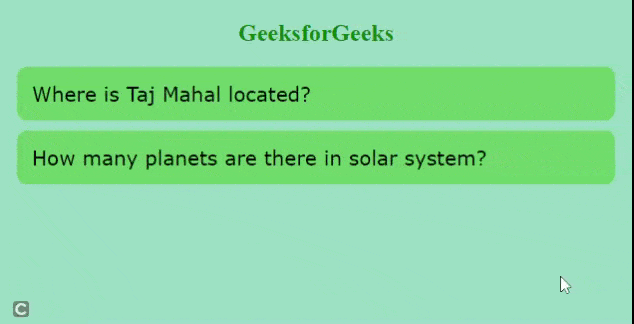# How to create an FAQ section to any website using JavaScript ?

• Last Updated : 20 Aug, 2021

In this article, we have created a Frequently Asked Questions(FAQ) accordion using JavaScript. The accordion is used to display the content in list format. It can expand or collapse to display the content it contains.

Approach:

• Create a nested HTML div tag with class names containing the questions and answers.
• For styling, add some CSS properties like alignment, font size, padding, margin, etc.
• Using JavaScript, implement functions that display answer or accordion-item when the question is clicked.

HTML Code:  We have used classes for questions and answers. These classes are used for styling purposes.

## index.html

 ` ` `<``html``> ` ` `  `<``head``> ` `  ``<``link` `rel``=``"stylesheet"` `href``=``"styles.css"``> ` `  ``<``script` `src``=``"main.js"``> ` ` ` ` `  `<``body``> ` `  ``<``h2` `style``=``"color:green; text-align:center"``> ` `    ``GeeksforGeeks ` `  `` ` `  ``<``div` `class``=``"layout"``> ` `    ``<``div` `class``=``"accordion"``> ` `      ``<``div` `class``=``"accordion__question"``> ` `        ``<``p``>Where is Taj Mahal located? ` ` `  `      `` ` `      ``<``div` `class``=``"accordion__answer"``> ` `        ``<``p``>Taj Mahal is located in Agra, Uttar Pradesh. ` `      `` ` `    `` ` ` `  `    ``<``div` `class``=``"accordion"``> ` `      ``<``div` `class``=``"accordion__question"``> ` `        ``<``p``>How many planets are there in solar system? ` `      `` ` ` `  `      ``<``div` `class``=``"accordion__answer"``> ` `        ``<``p``> ` `          ``There are eight planets in solar system. ` `          ``Mercury, Venus, Earth, Mars, Jupiter, Saturn, ` `          ``Uranus, and Neptune. ` `        `` ` `      `` ` `    `` ` `  `` ` ` ` ` `  ` `

CSS Code: For styling, we have used CSS properties.

## style.css

 `body { ` `  ``background-color``: ``rgb``(``153``, ``218``, ``196``); ` `} ` `.layout { ` `  ``width``: ``600px``; ` `  ``margin``: ``auto``; ` `} ` `.accordion { ` `  ``padding``: ``10px``; ` `  ``margin-top``: ``10px``; ` `  ``margin-bottom``: ``10px``; ` `  ``background``: ``rgb``(``105``, ``206``, ``105``); ` `  ``border-radius: ``10px``; ` `} ` `.accordion__question p { ` `  ``margin``: ``5px``; ` `  ``padding``: ``0``; ` `  ``font-family``: ``Verdana``; ` `  ``font-size``: ``20px``; ` `} ` `.accordion__answer p { ` `  ``margin``: ``5px``; ` `  ``padding``: ``10px``; ` `  ``font-size``: ``large``; ` `  ``font-family``: ``Verdana``, Geneva, ``Tahoma``, ``sans-serif``; ` `  ``color``: ``rgb``(``255``, ``255``, ``255``); ` `  ``background``: ``rgb``(``82``, ``170``, ``122``); ` `  ``border-radius: ``10px``; ` `} ` `.accordion:hover { ` `  ``cursor``: ``pointer``; ` `} ` `.accordion__answer { ` `  ``display``: ``none``; ` `} ` `.accordion.active .accordion__answer { ` `  ``display``: ``block``; ` `}`

Note: We can also create another file for styling or we can add them in the same HTML file under the style tag.

JavaScript code: We will use JavaScript to display the accordion when it is clicked. We have used the querySelectorAll() method to return a collection of elements that match the class name passed to this method and store these elements in variable answers.

We have used a forEach() method to call the function over each element in the answer variable. Now, specify an event listener addEventListener(), when the element is clicked. It will check if the event has an active class by using the classList property which returns the names of all the classes associated with that element or event.

If it has the active class, that means it is showing the answer or accordion is in an expanded state, then we will remove the active class from that event to hide the content. If it does not have an active class, that means the accordion is in the collapsed state, then we will add an active class.

## main.js

 `let answers = document.querySelectorAll(``".accordion"``); ` `answers.forEach((event) => { ` `  ``event.addEventListener(``"click"``, () => { ` `    ``if` `(event.classList.contains(``"active"``)) { ` `      ``event.classList.remove(``"active"``); ` `    ``} ``else` `{ ` `      ``event.classList.add(``"active"``); ` `    ``} ` `  ``}); ` `});`

Complete Code: In this section, we will combine all the codes together to create the FAQ

## HTML

 ` ` `<``html` `lang``=``"en"``> ` `<``head``> ` `    ``<``title``>FAQ ` `    ``<``style``> ` `        ``body{ ` `            ``background-color: rgb(153, 218, 196); ` `        ``} ` `        ``.layout{ ` `            ``width: 600px; ` `            ``margin: auto; ` `        ``} ` `        ``.accordion{ ` `            ``padding: 10px; ` `            ``margin-top: 10px; ` `            ``margin-bottom: 10px; ` `            ``background: rgb(105, 206, 105); ` `            ``border-radius: 10px; ` `        ``} ` `        ``.accordion__question p{ ` `            ``margin: 5px; ` `            ``padding: 0; ` `            ``font-family: Verdana; ` `            ``font-size: 20px; ` `        ``} ` `        ``.accordion__answer p{ ` `            ``margin: 5px; ` `            ``padding: 10px; ` `            ``font-size: large; ` `            ``font-family: Verdana, Geneva, Tahoma, sans-serif; ` `            ``color: rgb(255, 255, 255); ` `            ``background: rgb(82, 170, 122); ` `            ``border-radius: 10px;  ` `        ``} ` `        ``.accordion:hover{ ` `            ``cursor: pointer; ` `        ``} ` `        ``.accordion__answer { ` `            ``display: none; ` `        ``}  ` `        ``.accordion.active .accordion__answer { ` `            ``display: block; ` `        ``} ` `    `` ` ` ` `<``body``> ` `    ``<``h2` `style``=``"color:green; text-align:center"``>GeeksforGeeks ` `    ``<``div` `class``=``"layout"``> ` `        ``<``div` `class``=``"accordion"``> ` `            ``<``div` `class``=``"accordion__question"``> ` `                ``<``p``>Where is Taj Mahal located? ` ` `  `            `` ` `            ``<``div` `class``=``"accordion__answer"``> ` `                ``<``p``>Taj Mahal is located in Agra, Uttar Pradesh. ` ` `  `            `` ` `        `` ` `        ``<``div` `class``=``"accordion"``> ` `            ``<``div` `class``=``"accordion__question"``> ` `                ``<``p``>How many planets are there in solar system? ` ` `  `            `` ` `            ``<``div` `class``=``"accordion__answer"``> ` `                ``<``p``> ` `                  ``There are eight planets in solar system.  ` `                  ``Mercury, Venus, Earth, Mars, Jupiter, Saturn,  ` `                  ``Uranus, and Neptune. ` `                `` ` ` `  `            `` ` `        `` ` `    `` ` ` `  `    ``<``script``> ` `        ``let answers=document.querySelectorAll(".accordion"); ` `        ``answers.forEach((event)=>{ ` `            ``event.addEventListener('click',()=>{ ` `                ``if(event.classList.contains("active")){ ` `                    ``event.classList.remove("active"); ` `                ``} ` `                ``else{ ` `                    ``event.classList.add("active"); ` `                ``} ` `            ``}) ` `        ``}) ` `    `` ` ` ` ``

Output:FAQ feature

My Personal Notes arrow_drop_up
Recommended Articles
Page :